Olympiad Test: Number Ranking - 1

# Olympiad Test: Number Ranking - 1

Test Description

## 15 Questions MCQ Test Science Olympiad Class 8 | Olympiad Test: Number Ranking - 1

Olympiad Test: Number Ranking - 1 for Class 8 2023 is part of Science Olympiad Class 8 preparation. The Olympiad Test: Number Ranking - 1 questions and answers have been prepared according to the Class 8 exam syllabus.The Olympiad Test: Number Ranking - 1 MCQs are made for Class 8 2023 Exam. Find important definitions, questions, notes, meanings, examples, exercises, MCQs and online tests for Olympiad Test: Number Ranking - 1 below.
 1 Crore+ students have signed up on EduRev. Have you?
Olympiad Test: Number Ranking - 1 - Question 1

### How many odd numbers are there in the sequence each of which is immediately followed by an odd number? 5 1 4 7 3 9 8 5 7 2 6 3 1 5 8 6 3 8 5 2 2 4 3 4 9 6

Detailed Solution for Olympiad Test: Number Ranking - 1 - Question 1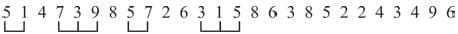Olympiad Test: Number Ranking - 1 - Question 2

### How many odd numbers are there in the Sequence which are immediately preceded and also immediately followed by an even number in the sequence ? 5 1 4 7 3 2 5 6 8 9 6 7 3 2 1 5 6 4 3 2 7 4

Detailed Solution for Olympiad Test: Number Ranking - 1 - Question 2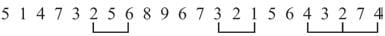Olympiad Test: Number Ranking - 1 - Question 3

### How many even numbers are there which are immediately followed by an odd number and also immediately preceded by an odd number in the sequence? 8 4 7 6 5 3 2 5 1 6 4 3 2 6 7 9 8 5

Detailed Solution for Olympiad Test: Number Ranking - 1 - Question 3Olympiad Test: Number Ranking - 1 - Question 4

In the series
2 5 3 4 8 7 4 2 6 7 1 5 8 3 7 4 5 3
How many pair of successive numbers have a difference of 3?

Detailed Solution for Olympiad Test: Number Ranking - 1 - Question 4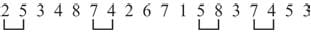Olympiad Test: Number Ranking - 1 - Question 5

How many 5’s are there in the sequence which are immediately preceded by 4 and immediately followed by 7?
2 3 4 5 6 1 4 5 7 1 2 4 5 7 3 8 4 5 9 6 5 3

Detailed Solution for Olympiad Test: Number Ranking - 1 - Question 5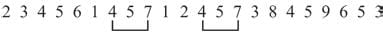Olympiad Test: Number Ranking - 1 - Question 6

How many pair of successive numbers are in the given series whose sum is 12?
4 5 7 3 2 8 4 9 1 5 6 4 8 3 1 9 3 5 7

Detailed Solution for Olympiad Test: Number Ranking - 1 - Question 6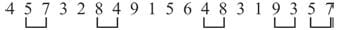Olympiad Test: Number Ranking - 1 - Question 7

How many pair of successive numbers ar ein the given series whose product is an even number?
6 1 4 6 3 5 1 7 2 6 3 5 7 1 8 3 7 5

Detailed Solution for Olympiad Test: Number Ranking - 1 - Question 7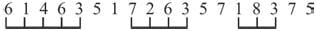Olympiad Test: Number Ranking - 1 - Question 8

How many 9’s are there in the series which are preceded by 7 but not followed by 8?
4 2 9 6 7 9 2 4 7 9 8 4 7 9 3 6 8 9

Detailed Solution for Olympiad Test: Number Ranking - 1 - Question 8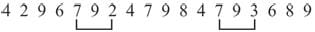Olympiad Test: Number Ranking - 1 - Question 9

What is the product of sum of even digits and sum of odd digits in the number
8 6 7 4 3 5 2?

Detailed Solution for Olympiad Test: Number Ranking - 1 - Question 9

Sum of even digits = 8 + 6 + 4 + 2 = 20
Sum of odd digits = 7 + 3 + 5 = 15
Product = 20 × 15 = 300

Olympiad Test: Number Ranking - 1 - Question 10

How many 8’s are there in the series which are preceded by 5 but not followed by 7?
4 3 8 6 5 8 2 3 5 8 4 7 5 8 7 4

Detailed Solution for Olympiad Test: Number Ranking - 1 - Question 10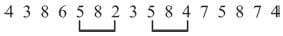Olympiad Test: Number Ranking - 1 - Question 11

What is the difference between the sum of even digits and sum of odd digits in the number
7 4 6 2 5 8 9 3?

Detailed Solution for Olympiad Test: Number Ranking - 1 - Question 11

Sum of even digits = 4 + 6 + 2 + 8 =20
Sum of odd digits = 7 + 5 + 9 + 3 = 24
Difference = 24 – 20 = 4.

Olympiad Test: Number Ranking - 1 - Question 12

If the given series is written in the reverse order which number will be 5th to the right of the seventh number from the left?
7, 3, 8, 7, 0, 3, 4, 6, 2, 5, 9, 6, 4, 7

Detailed Solution for Olympiad Test: Number Ranking - 1 - Question 12

Given series 7, 3, 8, 7, 0, 3, 4, 6, 2, 5 , 9, 6, 4, 7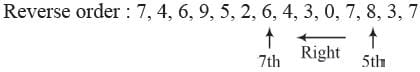Olympiad Test: Number Ranking - 1 - Question 13

In the given number sequence, how many such even numbers which are exactly divisible by its immediate preceding number but not exactly divisible by its immediate succeeding number?
3 5 6 4 8 7 1 2 4 3 6 4 5 2 6 7

Detailed Solution for Olympiad Test: Number Ranking - 1 - Question 13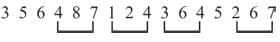Olympiad Test: Number Ranking - 1 - Question 14

From the given numbers
823, 716, 539, 683, 937
what will be the first digit of the second highest number after the position of only second and third digits with in each number are interchanged ?

Detailed Solution for Olympiad Test: Number Ranking - 1 - Question 14

Given numbers – 823, 716, 539, 683, 937
New numbers – 832, 761, 593, 638, 973
Second highest number = 832.
Ist first digit = 8

Olympiad Test: Number Ranking - 1 - Question 15

From the given numbers
728, 593, 645, 296, 968
what will be the first digit of the second highest number after the position of only first and third digits within each number are
interchanged?

Detailed Solution for Olympiad Test: Number Ranking - 1 - Question 15

Given numbers – 728, 593, 645, 296, 968
New numbers – 827, 395, 546, 692, 869
Second highest number = 827
Ist first digit = 8